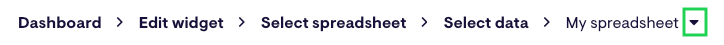Spreadsheet widgets powered by import functions, such as ImportRange and ImportData, automatically refresh around every 15 minutes. If this isn't fast enough for your needs, you can set your own refresh interval by writing a script using Google Apps Script Editor.

Be aware that when data from multiple import functions – each with their own refresh intervals – are used in the same connected spreadsheet, it can affect the accuracy of the data displayed.

## ImportData function script

To write a script for the ImportData function, follow these steps:

1. Click on the Tools menu and select the option Script Editor.
2. Replace the default function with following script. Make sure the cell in your Sheet exactly match the one in the script, otherwise ImportData will not update automatically.
``````function myFunction() {
}``````## ImportHTML function script

To write a script for the ImportHTML function, follow these steps:

1. In a new Google Sheet, select the Tools menu and click Script Editor. A new Google Apps Script tab will open.
2. Replace the default code with the following script:
``````function getData() {
var queryString = Math.random();
var cellFunction = '=IMPORTHTML("<url>?","table",<index>)';

sheetName.getRange('<import-cell>').setValue(cellFunction);
}``````
3. Replace `<sheet-name>` with the name of your sheet (the tab name, not the name of the file).
4. Replace `<url>` with the URL of your web page, e.g. `https://www.bloomberg.com/markets/currencies/cross-rates`.
5. Replace `<index>` with the table position on the page, e.g. `1`.
6. Replace `<import-cell>` with the cell where you want to put your import statement, e.g. `A1`.8. Select File, then choose Save. Then skip to setting up a trigger for your new script.

If you're curious about why the script is adding a random number to the end of our URL - without it, the website will be cached and the function stops importing the latest data.

## ImportRange function script

To write a script for the ImportRange function, follow these steps:

1. Click on the Tools menu and select the option Script Editor.
2. Replace the default function with following script. Then edit the script by first replacing the `.setValue('IMPORTRANGE')` function with your own, then making sure the `.getRange('cell')` in your Sheet exactly match the one in the script, otherwise ImportRange will not update automatically.
``````function myFunction() {

}``````

## ImportXML function script

To write a script for the ImportXML function, follow these steps:

1. In a new Google Sheet, the first thing we'll do is split the ImportXML function into two logical pieces; the URL and the XPath query. Write the URL in cell A1 and the XPath query in cell A2.
2. If you want to add another ImportXML function into this sheet so that you can do calculations with the data, add the second URL in cell A4 and the XPath query in cell A5.
3. Give your Google Sheet a name.4. To write a script, click on the Tools menu.
5. Select the option Script Editor.
6. A Google Apps Script window will appear. Click the Blank Project option.
7. Replace the default function with the script shown below:
``````function getData() {
var queryString = Math.random();

var cellFunction1 = '=IMPORTXML("' + SpreadsheetApp.getActiveSheet().getRange('A1').getValue() + '?' + queryString + '","'+ SpreadsheetApp.getActiveSheet().getRange('A2').getValue() + '")';

var cellFunction2 = '=IMPORTXML("' + SpreadsheetApp.getActiveSheet().getRange('A4').getValue() + '?' + queryString + '","'+ SpreadsheetApp.getActiveSheet().getRange('A5').getValue() + '")';
}``````
8. Double-check the following aspects of the first section of the script (`var cellFunction1`...):

Cell reference C1 should reflect where you want to display the result of your ImportXML function.

9. If you want to include a second ImportXML function, double-check the following in the second section of the script (`var cellFunction2`...):

Cell reference C2 should reflect where you want to display the result of your second ImportXML function.

10. If you don't want to include a second ImportXML function, delete everything from `var cellFunction2...` to `...setValue(cellFunction2);` leaving just the following script:
``````function getData() {
var queryString = Math.random();

var cellFunction1 = '=IMPORTXML("' + SpreadsheetApp.getActiveSheet().getRange('A1').getValue() + '?' + queryString + '","'+ SpreadsheetApp.getActiveSheet().getRange('A2').getValue() + '")';

var cellFunction2 = '=IMPORTXML("' + SpreadsheetApp.getActiveSheet().getRange('A4').getValue() + '?' + queryString + '","'+ SpreadsheetApp.getActiveSheet().getRange('A5').getValue() + '")';
}
``````
11. Save your script, give it a name, then skip to setting up a trigger for your new script.In case you're wondering why we're adding a random number to the end of our URL, it's because otherwise the website will be cached and the function stops importing the latest data.

1. Click on the Tools menu and select the option Script Editor.
2. A Google Apps Script window will appear. Click the Blank Project option.
3. Replace the default function with the following script:
``function getData() { SpreadsheetApp.getActiveSheet().getRange('A2').setValue('=GOOGLEFINANCE("GOOG", "price")');}``

## Set up a trigger for your script

After writing your script set up a trigger so that it runs as often as you need.

1. From inside the Script Editor, click on the Edit menu and select the option Current project's triggers.
2. Click the + Add Trigger button in the bottom right corner of the page.3. Set a trigger for the myFunction function, that runs from Head on a Time-driven source that's triggered on a Minute timer.4. Once you set the trigger (and so that you don't have to wait 1 hour to get data), run the script for the first time.#### Tip

1. On the edit widget screen's breadcrumbs menu, click the downward arrow beside the name of your widget.2. Click , then your widget.